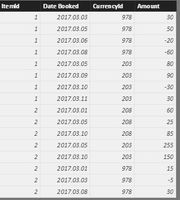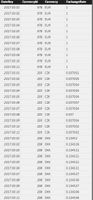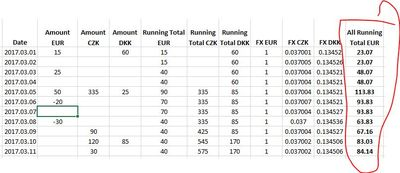cancel
Showing results for
Did you mean:Frequent Visitor

## Running Total multiple currency conversion to one

Hello

I have such simplified data model - with various currencies. I have to covert all those currencies in one  - running total EUR for each date in dimCalendar tabledata Model

facttable contains , ItemId, Date, CurrencyID & amountcurrencyExchange table contains Date, CurrencyId & exchange ratedimCurrencyExchange

result what I whant get would be like this (as measure not calculated column, this only to understand calculation, that each currency running total is converted to EUR and then summed together)result

currently I have created measure, which partly solves this problem (it is working while there is no in one date multiple transactions with different currencies) ([Total] is defined as SUM(fctTable[Amount])

```Running Total All EUR:=SUMX(FILTER(ALL(dimCalendar);dimCalendar[DateKey]<=MAX(dimCalendar[DateKey]));[Total]*
LOOKUPVALUE(
dimCurrencyExchange[ExchangeRate];
dimCurrencyExchange[DateKey];CALCULATE(VALUES(dimCalendar[DateKey]));
dimCurrencyExchange[CurrencyId];CALCULATE(VALUES(fctTable[CurrencyId]))))```

What could be the solution to get appropriate currency rate to given dates if there is no transactions, but running total would return value?

1 ACCEPTED SOLUTIONSuper User

@nauriso1

Apologies, missed that detail.

So the running total of transactions in each local currency is revalued daily in EUR at the current rate.

This measure will do the trick in the model you uploaded:

```Running Total EUR =
SUMX (
dimCurrency,
[Running Total]
* CALCULATE (
VALUES ( dimCurrencyExchange[Exchange Rate] ),
LASTDATE ( dimCalendar[Date Key] )
)
)```

The LASTDATE(...) is just there to ensure correct calculation at a total level when multiple dates are selected.

Model uploaded with new measure here for reference

Cheers,

Owen

Owen Auger
Blog
8 REPLIES 8Super User

I would tweak the data model & measures slightly to get this to work:

Sample model here

First of all, I would suggest you add a dimCurrency table to your data model, which contains a unique list of currency IDs/Names, and relate dimFX and fctTable to dimCurrency.

So you end up with fctTable & dimFX both related to dimCalendar & dimCurrency:Then I would define the measures below. [Amount EUR] iterates over the combinations of Date/Currency present in fctTable and uses relationships to look up the exchange rate (at Date/Currency granularity):

```Amount Local Currency =
SUM ( fctTable[Amount] )

Amount EUR =
SUMX (
SUMMARIZE ( fctTable, dimCalendar[DateKey], dimCurrency[CurrencyId] ),
// Note: VALUES ( dimFX[ExchangeRate] ) is safe because    // dimFX[ExchangeRate] has exactly one value for any DateKey/CurrencyId combination    CALCULATE (
VALUES ( dimFX[ExchangeRate] )
)
* [Amount Local Currency]
)

Amount EUR Cumulative =
CALCULATE (
[Amount EUR],
DATESBETWEEN ( dimCalendar[DateKey], BLANK (), MAX ( dimCalendar[DateKey] ) )
)```

Then the output looks like this:Variations on this are possible, but this seems a good way of doing it to me.

You could also pre-calculate [Amount EUR] in fctTable either in the Query Editor or with DAX.

Hopefully that is of some use.
Cheers,

Owen 🙂

Owen Auger
BlogFrequent Visitor

Thanks @OwenAuger for your replay, but I need, that running total is converted to EURos not transactions. In your case at first are transactions converted to EUR and then running total is made, which is not correct (because exchange rate used for running total is based on transaction date exchange rate, but I need that for running total would be applied appropriate date exchange rate regardless of transaction date.. So thats mean, tad running total should be converted by each date exchange rate)
I attached excel and pbx file. Hope that this will more clarify what I want achieve

RunningTotalCurrency_Conversion.pbix

RunningTotalCurrency_Conversion.xlsxSuper User

@nauriso1

Apologies, missed that detail.

So the running total of transactions in each local currency is revalued daily in EUR at the current rate.

This measure will do the trick in the model you uploaded:

```Running Total EUR =
SUMX (
dimCurrency,
[Running Total]
* CALCULATE (
VALUES ( dimCurrencyExchange[Exchange Rate] ),
LASTDATE ( dimCalendar[Date Key] )
)
)```

The LASTDATE(...) is just there to ensure correct calculation at a total level when multiple dates are selected.

Model uploaded with new measure here for reference

Cheers,

Owen

Owen Auger
BlogNew Member

Thanks, very useful and elegant solution 🙂Frequent Visitor

Thanks @OwenAuger! Could you, please, explain, what is happening "behind the scenes"?Super User

@nauriso1

Sure.

To put the Running Total EUR measure in words:

For each currency, calculate the Running Total in that currency, then multiply that by the Exchange Rate as at the date selected (or last date if multiple dates are selected).

Or describing what the DAX is doing:

1. SUMX iterates over each currency
2. For each currency, the [Running Total] measure returns the Running Total at the currently selected date (or max date)
3. This is multiplied by VALUES ( dimCurrencyExchange[Exchange Rate] ), calculated in the context of LASTDATE ( dimCalendar[Date Key] ) which should just have one value.
4. Since the VALUES(...) from Step 3 is wrapped in a CALCULATE, context transition means the current Currency is added to the filter context, and filters the dimCurrencyExchange table appropriately.

One potential performance issue is that we are iterating over all currencies, regardless of whether they have had transactions so far. If it is likely that they all have, then this doesn't matter.

Otherwise, you may consider iterating over just those currencies that have occurred so far, but I would test performance to see if it is worth it. Something like this:

```Running Total EUR =
SUMX (
CALCULATETABLE (
CALCULATETABLE ( dimCurrency, fctTable ),
DATESBETWEEN ( dimCalendar[Date Key], BLANK (), MAX ( dimCalendar[Date Key] ) )
),
[Running Total]
* CALCULATE (
VALUES ( dimCurrencyExchange[Exchange Rate] ),
LASTDATE ( dimCalendar[Date Key] )
)
)```

Cheers,

Owen

Owen Auger
BlogHelper II

I was truly amazed with the elegant solution and trying to use it to solve my challenge. I've recreated the original solution in Excel and it works fine. However modifying it for my own challenge I can't make it work. I have to apply 2 different FX rates for the same month - one (average) for P&L accounts and another (closing) to the Balance sheet ones (therefore my currencies expanded to 4 letters - USDF. USDB etc, where F  stands for flow, B is for Balance). I still have just one combination for currency and date in the currency exchange table, but my Running Total in CAD returns blanks. Can you please have a look at my attempt below?

Example

Cheers,Super User

Apologies for taking a while to get back to you.

The structure of your data model seems fine as far as relationships and having the B/F variants of each currency.

Regarding the blank Running Total CAD measure:

When I created a simple PivotTable with your model it looked like thisThe Running Total CAD measure does return values as long as there is a value defined in dimCurrencyExchange for the relevant date. However, your CADB values end at 8/1/2018, so you get blank running totals after that date. (When you view the measure value in the PowerPivot window, it displays the measure in an unfiltered date context).

You may also want to consider wrapping running total measures in an IF to check whether the date has gone past the last transaction date, and if so blank out the measure. Example on DAX Patterns here

Cheers,

Owen

Owen Auger
BlogAnnouncements#### Exclusive opportunity for Women!

Join us for a free, hands-on Microsoft workshop led by women trainers for women where you will learn how to build a Dashboard in a Day!#### Power Platform Conference-Power BI and Fabric Sessions

Join us Oct 1 - 6 in Las Vegas for the Microsoft Power Platform Conference.Top Solution Authors
Top Kudoed Authors
Users online (3,007)